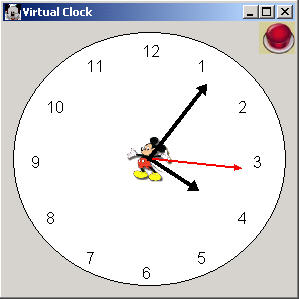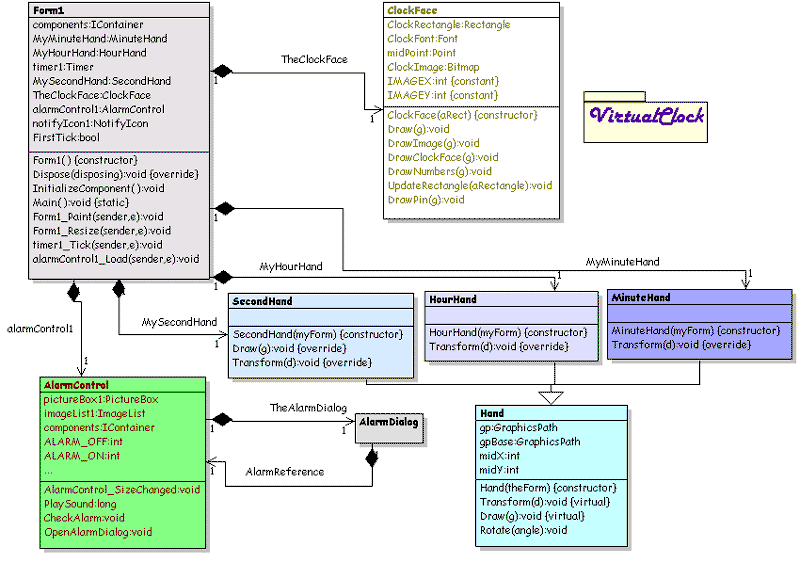# A Virtual Clock in C# and GDI+Fig 1 - Virtual Clock

It's that time again!  Time to check out a new project written C# and the .NET library. This article describes how to create a virtual clock in C#.  The hands of the clock are drawn using GraphicPaths. The UML Design for the Virtual Clock is shown below:Fig 2 - UML Design of the Virtual Clock reverse engineered using WithClass 2000

The design is a simple Composite of Hands and a ClockFace. The Hand class serves as a base class for the HourHand, MinuteHand, and SecondHand,  each of which have their own GraphicsPath instance stored in the base class.  Each time the timer ticks 1 second, the hands are redrawn according to the current time.  The sequence of events are shown below:Fig 3 - One Second Timer Tick Sequence Diagram drawn in WithClass

The Transform method takes the DateTime(which is actually the current time) and rotates the GraphicsPath of the clock hand by the corresponding Time component for the particular hand.  Since the time component is different for each hand, the Transform Method is overridden for each hand.  Below is the transform method for the MinuteHand:

public override void Transform(DateTime d)
{
// turn the datetime minute component into an angle
// remember: 60 seconds in 2 pi (1 circle)
// Also note that we need to add the fractional seconds component to get the most accuracy
double minuteTime = (double)d.Minute + (double)(d.Second/60.0);
double angle = ((double)minuteTime /60.0 ) * 360; // 2.0 * Math.PI;
// Rotate the graphics path
Rotate(angle);
}

The Rotation of the GraphicsPath is performed in the base class, because it's the same for all Hands on the Clock.  Rotation of a GraphicsPath is done through a Matrix.  (See previous articles mentioned above to see how this is done mathematically.)  The code for the rotation of the hands is shown below:

public void Rotate(double angle)
{
// Create a copy of the Graphics Path from the 0 degree path
gp = (GraphicsPath)gpBase.Clone();
// create a new transformation matrix
Matrix mTransform = new Matrix();
// rotate the matrix around the midpoint of the clock at the angle passed in.
mTransform.RotateAt((float)angle, new PointF(midX, midY));
// Transform the Graphics Path (which is actually the clock hand)
gp.Transform(mTransform);
}

The other noteworthy code you might find interesting is the drawing of the clock face.  The clock face is drawn by the ClockFace class.  This class draws the circle for the clock as well as the numbers around the clock and the image inside the clock:

public void Draw(Graphics g)
{
// draw blank face of clock
DrawClockFace(g);
// Draw clock Image
DrawImage(g);
// draw numbers around the face
DrawNumbers(g);
// draw clock pin
DrawPin(g);
}

The Numbers on the clock are drawn using the sine and cosine functions to determine the numeral positions:

private void DrawNumbers(Graphics g)
{
// initialize the count of the numbers around the clock
int count = 1;
// cycle around the circle on the circle on the clock and draw each number in the correct position
for (double a = 0; a < 2 * Math.PI; a += (2.0* Math.PI/12))
{
// calculate the x position of the next number
double x = (ClockRectangle.Width - 70)/2 * Math.Cos(a - Math.PI/3) + (ClockRectangle.Width - 70)/2 + 25;
// calculate the y position of the next number
double y = (ClockRectangle.Width - 70)/2 * Math.Sin(a - Math.PI/3)+ (ClockRectangle.Width - 70)/2 + 20;
// Draw the next Number according to the calculated position around the inner circumference of the circle
g.DrawString(Convert.ToString(count), ClockFont, Brushes.Black, (float)x,(float)y, new StringFormat());
// Increment the next Number
count++;
}
}

Improvements:

This clock could use one of those little date squares on the right side showing the day of the week. Also an alarm wouldn't be bad <g>.  Maybe on the next go around of the virtual clock. Time's up!